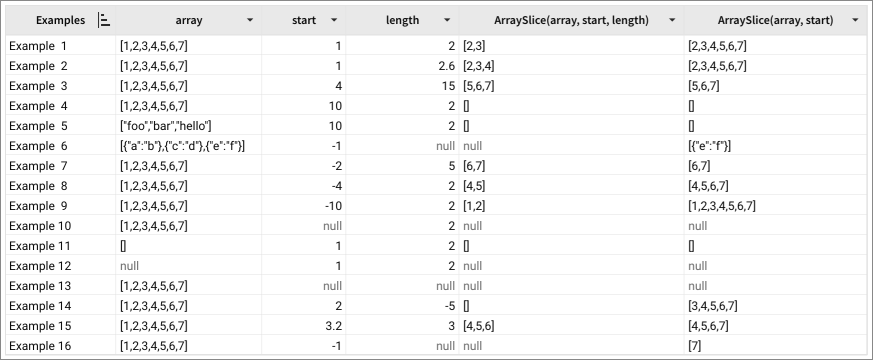# ArraySlice

The ArraySlice function returns a portion of an array, defined by the starting index and length.

Supported only on Snowflake, PostgreSQL, and BigQuery connections.

## Syntax

`ArraySlice(array, start, length)`

The ArraySlice function has the following arguments.

In general, if any of the arguments used by the function is `Null`, it returns `Null`.

array
Required.
The input array that Sigma parses to extract a portion as a new array.
Must be an array structure; to create single array from a column, use the ArrayAgg function.
If this value is `Null`, then Sigma does not return an array; see Example 12.
If the array is empty, Sigma returns an empty array; see Example 11.
start
Required.
The beginning, or the starting index for extracting the new array from the original array.
The range of valid valued are from `0` to `ArrayLength(array) - 1`.
If the value calculates beyond the end of the original array, Sigma returns an empty array, represented as `[]`. See Example 4, Example 5, and Example 11.
Negative values count from the end of the array, so `-2` is the second from the last position in the original array.
If this value is negative and the index calculates beyond the beginning of the original array, then the value `start` defaults to `0`. See Example 9.
If this value is negative and Sigma applies a `Null` `length` value, it does not return an array; see Example 6, column ArraySlice(array, start, length).
If both `start` and `length` are `Null`, the function does not return an array; it evaluates to `Null`. See Example 13.
Sigma rounds floating numbers to the nearest integer; see Example 15, where the applied start is `3`.
length
Optional.
The length of the new array.
If there is no value (`Null`) and Sigma applies it, then it does not return an array; see Example 6, column ArraySlice(array, start, length)
If the value is so large that the ending index calculates beyond the size of the original array, the function extracts from the start index to the end of the original array. See Example 3.
If this value is negative, the function returns an empty array; see Example 14.
If both `start` and `length` are `Null`, the function does not return an array; it evaluates to `Null`. See Example 13.
Sigma rounds floating numbers to the nearest integer; see Example 2, where the applied length is `3`.

## Examples

The following examples of ArraySlice function demonstrate how this function works.